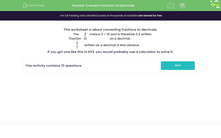# Convert Fractions to Decimals

In this worksheet, students will practise converting fractions to decimals.Key stage:  KS 3

Curriculum topic:   Number

Curriculum subtopic:   Work Interchangeably with Terminating Decimals and Fractions

Popular topics:   Percentages worksheets, Decimals worksheets

Difficulty level:#### Worksheet Overview

In this activity, we are going to look at converting fractions to decimals.

This is an important skill when we want to compare fractions and decimals.

The fraction

 2 10

means 2 ÷ 10 and is therefore 0.2 written as a decimal.

The fraction

 2 7

written as a decimal is less obvious.

To convert this fraction we can use the bus stop method or use a calculator.

We have to do the calculation 2 ÷ 7 = 0.286 to 3 decimal places.

Let's try some questions.

### What is EdPlace?

We're your National Curriculum aligned online education content provider helping each child succeed in English, maths and science from year 1 to GCSE. With an EdPlace account you’ll be able to track and measure progress, helping each child achieve their best. We build confidence and attainment by personalising each child’s learning at a level that suits them.

Get started